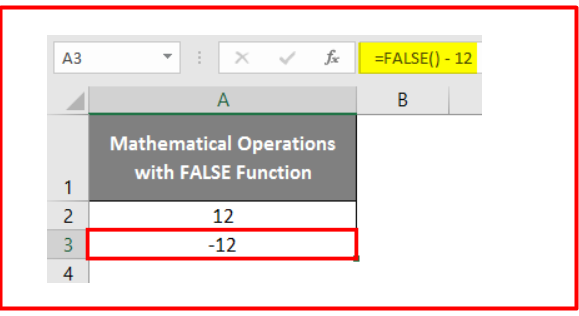# How to Create a True False Function in Excel? [With Images]

To create a True False function in Excel, you’ll need to use the logical operators TRUE and FALSE. These operators return a value of TRUE or FALSE depending on the conditions you specify.

Contents

## What is True Function in Excel?

True function in Excel is a way to ensure that a specific cell always contains the most up-to-date information from another cell or cells. This is done by creating a formula that references the cell or cells that you want to keep track of. The true function will then automatically update the cell you designated as containing the most recent information. It is extremely useful for keeping large and complex data sets organized and up-to-date.

## What is the True Function Formula?

The True Function Formula is a simple mathematical formula that you can use to calculate the absolute value of a number.

True Function Formula

How to Create a True Function in Excel?

To create a true function in Excel, you must first select a cell in the worksheet. Then, click the Formulas tab on the ribbon and select the function you want to use from the Function Library.

Excel will insert the function into the cell, and you can then enter the arguments for the function.

Here I share three different methods to create a true function in Excel.

### Method 1: True Function with If Function

Now we will see the use of a TRUE function with an IF function. We want to check if the below values are greater than 30.

For this example, I use the values 34, 12, 78, 45, 24, 56, and 10.

The syntax for the TRUE function is as follows: =TRUE().

For our first value, 35, we will get the following result: =IF(A12>30,TRUE()). And this results in a value of TRUE since 35 is greater than 30.

Drag and drop that formula to apply all the cells.

### Method 2: True Function with Logical Condition

If a function is true, it will return a logical value of true. If the function is false, it will return a logical value of false. You can write functions in a variety of ways, but they all must adhere to this basic rule to produce accurate results.

### Methos 3: True Function with BOOLEAN Function

When working with boolean functions, it is important to remember that these functions return a value of either true or false.

## What is a False Function in Excel?

The false function in Excel is a logical function that returns the value FALSE. You can use it to test for conditions that are not true. For example, if you have a cell with the value “TRUE” and want to test if it is not true, you would use the FALSE function.

## What is False Function Formula?

False Function Formula is a math error that can occur when calculating an object’s volume or surface area. The error happens when the formula used for the object’s dimensions is incorrect.

False Function Formula

## How to Create a False unction in Excel?

To create a false unction in Excel, select the cells to which you want to apply the function. Then, in the Home tab, click the drop-down arrow next to the false unction button and select the function you want to use. Excel will automatically apply the function to the selected cells.

Here I share three different methods to create a False function in Excel.

### Method 1: False Function with Logical Function

When using a logical function in Excel, it is important to remember that it will return a “FALSE” value if any logical conditions are not met. This can be frustrating if you expect the function to return a “TRUE” value, so it is important to check all the conditions carefully.

Step 1: Go to the Formula tab and click on the Logical function. And then select the False function.

Step 2:Click OK in the pop-up window that appears. Doing so will return the logical value FALSE as output in the working cell.

Step 3: To insert the logical FALSE value in your active sheet, type FALSE or =FALSE(). See the screenshot below for an example.

### Method 2: False Function for Logical Condition

If a logical condition is required to evaluate to false for a program to function correctly, then you can use a false function.

For example, here, I put 32 values on the A2 cell. And on cell B2 type, the following formula=A2<20.

Press the key Enter and see the result.

### Method 3: False Function with Mathematical Operation

You can use mathematical operations to create more complex criteria when using the FALSE function in Excel.

For example, type the formula in cell A2 of your active excel sheet.

=FALSE() +12 and press the Enter key. This will calculate the number of characters in the cell above it. You will receive an answer equivalent to (0 + 12). This means that your question has been answered correctly and completely.

In cell A3 of the active Excel sheet, type the following formula and press the Enter key:=FALSE() -12

The output will equal what you get from (0 – 12).I hope this article will help you create a true false function that will increase or decrease a cell’s value based on another cell’s data. In this example, the function would increase or decrease its value based on another cell’s value.